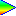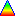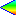# NAND-based boolean logic identities

Since the NAND gate is a universal gate (i.e. all other gates can be created from it), we could treat boolean logic as if it had only one operation (NAND). I searched around on the net for a set of identities for purely NAND-based boolean logic, but found nothing. So I've put them up here for reference.

## Notation

I assume that the only operation permitted is a two-input NAND operation. We can indicate NAND by simple concatenation (no operator between two arguments), but that still leaves us with a confusing number of parentheses, e.g. a((b1)(c1)). So to make it easier to read, I use the following notation:

• 0 and 1 are false and true
• Letters a to z are variables
• Parentheses enclose a subexpression
• A sequence of a power of two number of false/true/variables/subexpressions concatenated represent a balanced tree. For example: abcd is (ab)(cd), or abcdefgh is ((ab)(cd))((ef)(gh)). Due to the way that the two inputs of a NAND can be swapped, these sequences can be reordered only in certain ways, e.g. abcd = bacd = cdab, but abcd != cbad.
• A dot is a NAND operator at a lower precedence than concatenation. So we can write 1.ab instead of 1(ab)
• A space is a NAND operator at a lower precedence than dot. So we can write c 1.ab instead of c(1(ab))

I have a perl script downloadable HERE which evaluates expressions in this notation and produces truth tables, for example:

```\$ ./nand-eval.pl -l 'a.1(b.c1 c.b1) a1.(b.c1 c.b1)'
a b c   ((a(1((b(c1))(c(b1)))))((a1)((b(c1))(c(b1))))) (15 gates)
0 0 0    0
0 0 1    1
0 1 0    1
0 1 1    0
1 0 0    1
1 0 1    0
1 1 0    0
1 1 1    1
```

## Converting from NOT, AND, OR and XOR

Common operations such as AND, OR, XOR and NOT can be converted to NAND logic as follows (using C notation for bit-ops to avoid confusion):

```NOT: ~a -> a1

AND: a&b -> 1.ab
a&b&c -> 1(a 1.bc)
a&b&c&d -> 1(1.ab 1.cd)

OR:  a|b -> a1b1
a|b|c -> a1 1.b1c1
a|b|c|d -> 1.a1b1 1.c1d1

XOR: a^b -> a.b1 b.a1 or 1 ab.a1b1
a^b^c -> a.1(b.c1 c.b1) a1.(b.c1 c.b1)
a^b^c^d -> (a.b1 b.a1).1(c.d1 d.c1) 1(a.b1 b.a1).(c.d1 d.c1)
```

## Identities

Here are the identities, which I mostly derived by converting boolean AND/OR/NOT identities to NAND logic and reducing them down. Note that one identity often covers two AND/OR identities:

```  11 = 0
a0 = 1
aa = a1
ab = ba                   (exchange of two variables)
a.a1 = 1                  (equivalent to aa' and a+a')
a.ab = a.1b
1.1a = a                  (equivalent to a'' = a)

1 a.bc = a.b1 a.c1        (four forms of the same expression,
1.abac = a.b1c1            equivalent to a(b+c) and (a+b)(a+c)
abac = 1 a.b1c1            expansions)
a.bc = 1(a.b1 a.c1)

c 1.ab = a 1.bc = b 1.ac  (exchange of three variables: 3-input NAND)
1.ab 1.cd                 (abcd may be exchanged freely: 4-input NAND)
a 1.b(1.cd)               (abcd may be exchanged freely: 4-input NAND)
```

Have I missed anything?These pages and files, including applets and artwork, are Copyright (c) 1997-2019 Jim Peters unless otherwise stated. Please contact me if you'd like to use anything not explicitly released, or if you have something interesting to discuss.